Function Repository Resource:

# MatrixFormDivided

Add row and column dividing lines to MatrixForm

Contributed by: Gustavo Delfino
 ResourceFunction["MatrixFormDivided"][m,step] returns a MatrixForm of matrix m with divider lines every step columns and rows. ResourceFunction["MatrixFormDivided"][m,{xstep,ystep}] use different step specifications for colums and rows. ResourceFunction["MatrixFormDivided"][m,{{s1,s2,…,sn}}] uses divider lines with specified sizes s where each si represents a number of columns and rows. ResourceFunction["MatrixFormDivided"][m,{{sx1,sx2,…,sxn},{sy1,sy2,…,syn}}] uses diferent divider lines specific spacings for rows and columns. ResourceFunction["MatrixFormDivided"][spec] represents an operator form of ResourceFunction["MatrixFormDivided"] that can be applied to a matrix.

## Details and Options

ResourceFunction["MatrixFormDivided"] takes the option "DividersOffset", which specifies the offset used for the dividers. This can be an integer or a list of two integers representing different row and column offsets.

## Examples

### Basic Examples (3)

Place divider lines at every second column and row:

 In:=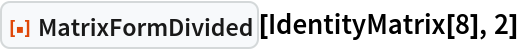Out=Place divider lines at every third column and row:

 In:=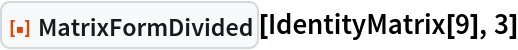Out=Use the operator form on a rectangular matrix:

 In:=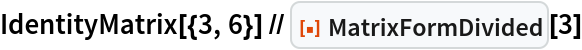Out=In:=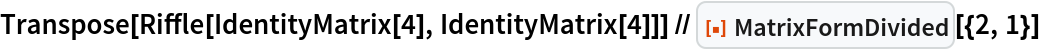Out=Using copy/paste or evaluate in place, it is possible to use MatrixFormDivided as part of input. This cannot be done with a Grid:

 In:=Out=### Scope (3)

The structure of composed matrices can be highlighted by using divider lines:

 In:=Out=In:=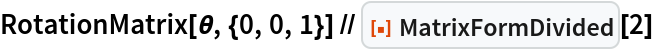Out=Use dividers at specific locations:

 In:=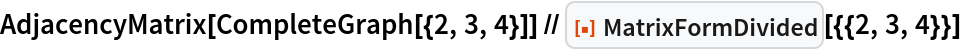Out=Use dividers at specific locations differently for rows and columns:

 In:=Out=### Options (3)

#### DividersOffset (3)

Place dividers for every two rows or columns, with an offset of one:

 In:=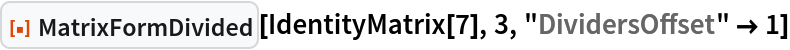Out=Use an offset of one only on the rows:

 In:=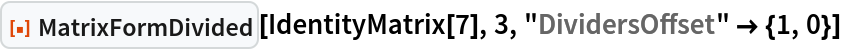Out=Use an offset of one only on the columns:

 In:=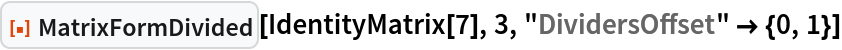Out=### Properties and Relations (1)

MatrixFormDivided is an extended version of MatrixForm:

 In:=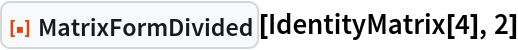Out=In:=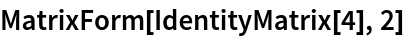Out=### Possible Issues (2)

The output of MatrixFormDivided is not directly computable:

 In:=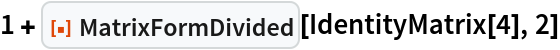Out=By evaluating it in place, it becomes computable:

 In:=Out=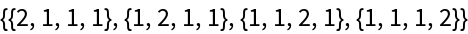The "DividersOffset" option has no effect when using specific spacings:

 In:=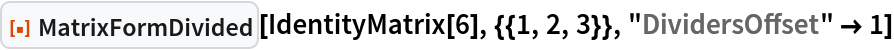Out=GustavoDelfino

## Version History

• 1.0.0 – 11 July 2022